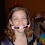## Tuesday, July 22, 2008

### A Whole Range of Numbers

If you multiply me by 2, subtract 1, and read the reverse the result you'll find me. Which numbers can I be?

1.Hint: Consider each number to be a polynomial of the form:
a(0)10^n + ... + a(n)10^0

Now we have well defined functions for what we wish to achieve (multiplication, addition and reversal of ordering).
Now we search for equations such that:
2*(a(0)10^n + ... + a(n)10^0 ) - 1 = a(n)10^n + ... + a(0)10^0
Rearranging and expanding gives:
(2a(0) - a(n))10^n + ... + (2a(n) – a(0))10^0 = 1
From which it becomes obvious that if n > 0 no solution can exist, thus assuming n = 0 we have
2a(0) – a(0) = 1
Therefore the polynomial 1 is the only solution to the problem.

2.You're on the right track. I may have made a mistake somewhere along the way, but 1 is not the only solution.

If we let the answer be x and let x = 10*A+ B (as in the A is the 10 digit and B is the 1 digit), then:
2*10*A+B - 1 = 10*B+A
19*A = 8*B + 1

8*B + 1 must be an odd number, therefore 19*A has to be an odd number.
The largest number 8*B + 1 can be is 73. Therefore 19*A <= 73. A must be 1, 2 or 3. A = 1 doesn't work, but A = 3 can work. If A is 3, then B = 7.

Therefore 37 is a possible solution.

You can then expand to the third digit and fourth digit and so on to get the possible solutions of:
37*2 - 1 = 73
397*2 - 1 = 793
3997*2 - 1 = 793
and so on....
399...997*2 - 1 = 799...993

3.Wow, great problem, Mike. Too bad I didn't check in yesterday...

4.Hi Mike, you're right - "From which it becomes obvious that if n > 0 no solution can exist" - am blaming a long day at the office for that appalling assumption on my part!!

5.I'm glad you guys enjoyed the question.

It's been tough lately trying to come up with new ones.

Leave your answer or, if you want to post a question of your own, send me an e-mail. Look in the about section to find my e-mail address. If it's new, I'll post it soon.

Please don't leave spam or 'Awesome blog, come visit mine' messages. I'll delete them soon after.

Enter your Email and join hundreds of others who get their Question of the Day sent right to their mailbox

The Lamplight Manor Puzz 3-D